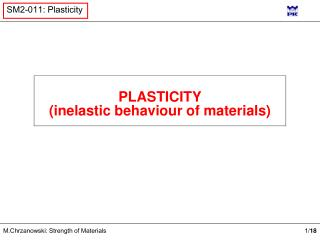DownloadDownload PresentationPLASTICITY (inelastic behaviour of materials)

# PLASTICITY (inelastic behaviour of materials)

Télécharger la présentation## PLASTICITY (inelastic behaviour of materials)

- - - - - - - - - - - - - - - - - - - - - - - - - - - E N D - - - - - - - - - - - - - - - - - - - - - - - - - - -
##### Presentation Transcript

1. PLASTICITY(inelastic behaviour of materials)

2. Rm RH  Re RH  Permanent plastic strain arctanE  Brittle material Linear elastic material Elastic materials when unloaded return to initial shape (strains caused by loading are reversible)  Plastic strains occurs when loads are high enough Elastoplastic material Plastic strains are irreversible

3.   RH Re Linear elasticity Stiff material with ideal plasticity Re   Elasticity with ideal plasticity    Re Re  Stiff material with plastic hardening Elasto-plastic material with plastic hardening   RH Typical real material  Different idealisations of tensile diagram for elasto-plastic materials

4.  z z Neutral axis zmax for for for Centre of gravity y x M A Elasto-plastic bending Elastic range Neutral axis Beam cross-section Side view

5. z’ y’ z zmax Centre of gravity z’ y A x’ Elasto-plastic bending Plastic limit moment Elastic limit moment Elastic neutral axis Plastic neutral axis

6. z’ z’ z’ N1 z1’ z2’ x’ y’ y’ N2 CoG ofA2 CoG of A1 Elasto-plastic bending Plastic limit moment A1 A A2

7. z’ z1’ z2’ y’ z y A Elasto-plastic bending Limit elastic moment Limit plastic moment A/2 A/2 k1 – shape coefficient

8. z z 2 2 b h b h ( ) h h = = = = W W 2 S A 2 b spr pl yc 1 6 2 4 4 A1 2 é ù b h æ ö ( ( ) ) h h h h = - = - - = ç ÷ W S A S A b b ê ú pl yo 1 yo 2 è ø h 2 4 2 4 4 ë û yc= yo 2 2 W b h b h M pl = = = = = M R M R k 1 . 5 A2 e e 6 4 W M spr b p 3 d = W spr 32 p 2 d 4 d 2 3 ( ) 1 d = = = W 2 S A 2 pl yc 1 p 2 4 3 6 W 32 pl = = = k 1 . 7 p W 6 spr A1 d yc= yo A2

9. 5 1 5 2 1 2 6 k = 1.76 2 2 2 1 a 3 Loading plane 15 k = 2.34 k = 1.52 20 9 k = 1.42 8 k = 1.45 10 k = 2.38 7 1 4 5 2 5 5 5 3 4 3 12 6 9 MC riddle: k=1,5 k=k(a)=? k=?

10.  Plastic limit of a cross-section Elasticity with ideal plasticity Statically determined No plastic gain Homogeneous distribution Non-homogeneous distribution Plastic gain Statically undetermined

11. a a 1 1 P Limit analysis of structures Statically determined structures Length and cross-section area of both bars: l, A Elastic solution  Plastic solution From equilirium: Stress in bars: In limit elastic state: Limit elastic capacity: Limit plastic capacity:

12. a a a P 1 1 2 Limit analysis of structures Statically undetermined structures Length and cross-section area of both bars: l, A Elastic solution Equilibriuim : Displacement compatibility:  Elastic limit capacity – plastic limit in bar #2 Plastic limit capacity – plastic limit in bars #1 and #2

13. 1,40 1,365 1,30 67,5o 1,20 1,10 a [o] 1,00 90 0 10 20 30 40 50 60 70 80 Capacity of the 3-bar structure due to plastic properties

14. z’ x’ Plastic hinge: Limit analysis of beams Concept of plastic hinge Trace of the cross-section plane according to the Bernoulli hypothesis Beam axis

15. Limit analysis of beams Moment–curvature interdependence In elastic range: In plastic range: k 1 1

16. Limit analysis of beams Plastic hinge Statically determined structures Bending moment Curvature Plastic zone spreading

17. Unstable mechanism! Limit elastic moment Limit plastic moment Shear forces diagram Statically indetermined structures

18. l/2 l/2 Limit analysis by virtual work principle In limit plastic state the moment distribution due to given mechanism is known. Example: On this basis limit plastic capacity can be easily found, however, the ratio of plastic to elastic capacity is unavailable. In a more complex case one has to consider all possible mechanisms. The right one is that which yields the smallest value of limit plastic capacity.

19. stop